## Year 5 Calculating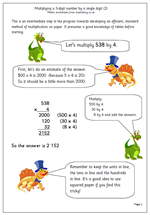There is a great deal expected of children in year 5 when it comes to calculating. Mental methods of calculating with whole numbers are extended to include addition of three small numbers, knowing tables and multiplying by 25. Efficient written methods of addition and subtraction of both whole numbers and decimals is expected as well as refining written methods of multiplication and division.

I have a growing range of worksheets covering these topics which can be found in the Year 5 Calculating category. Further worksheets on written methods can also be found in the Four Rules category.

Go to Year 5 calculating worksheets

## KS2 Maths 2010 Paper A Questions 9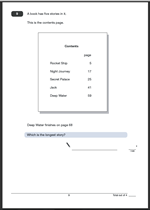Question 9, which is worth one mark for a correct answer, is much more of a level 4 question for several reasons.
It is a less straightforward question to answer as it is not immediately obvious what has to be done by just glancing at it. A further complication is that more information is given outside of the contents page, i.e. that Deep Water finishes on page 68. Some children will miss this fact and be confused as to how to work the answer out.
The most obvious way to do this is to treat it as a series of subtraction calculations;
Rocket Ship: 17 – 5 = 12
Night Journey: 25 – 17 = 8
Secret Palace: 41 – 25 = 16
Jack: 59 – 41 = 18
Deep Water: 68 – 59 = 9
Counting on is one way of doing this mentally and you may well see children using their fingers to count on whilst working this out.

Question 9 from SATs test 2010 Paper A

## Year 3 mental arithmetic: Sets 17 and 18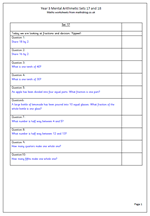Continuing with our weekly sets of mental arithmetic questions for Year 3 children, this week we concentrate on fractions and division. Not as hard as it sounds!

Many people these days think that as well as learning tables, children should learn their division facts. So they will know the 5 times table:

1 x 5 = 5, 2 x 5 = 10, 3 x 5 = 15 etc

but they should also know 15 divided by 5 = 3 etc.

The questions also look at finding tenths of numbers (divide by 10) and finding numbers which are half way between two consecutive numbers. Getting these correct will show a good understanding of number.

Year 3 Mental arithmetic (sets 17 and 18)

## Resource of the Week: Handling data wordsnake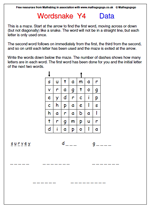This is known as a wordsnake, and is rather like a maze. Start at the arrow to find the first word, moving across or down, but not diagonally, rather like a snake. The word will not be in a straight line so might be quite tricky to find, although the first word (survey) has been given to make it a little easier.

The second word follows on immediately from the first; the third word from the second and so on until each letter in the grid has been used once. The last letter of the last word is where the arrow exits the maze.

Write the words down in the spaces provided. The number of dashes shows how many letters are in each word. The initial letters of the second and third words have also been given.

All the words in this wordsnake come from the vocabulary lists for Year 4 Handling Data.

This page and others similar can be found in the Tricky section of the Maths Puzzles and Board Games section of the site.

Year 4 Wordsnake: Handling Data vocabulary

## Year 2 Maths Worksheet: More missing numbers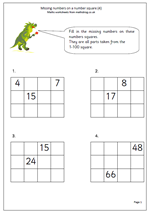The fourth, and probably last in a mini series of missing numbers on number grids. Each grid is part of a 1-100 number square with most of the numbers missing. The grid can be tackled in any order and it is interesting to see how confidently children approach this task. Some will be happiest by counting on in ones and filling in what they can before trying to count back in ones. Others will leap in and happily fill the columns in vertically, adding ten each time.

This and other similar pages can be found in the Year 2 Counting category.

Missing numbers on a number square (4)

## Maths worksheet: Division of money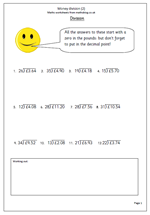I have had several requests to produce another division of money worksheet as it seems this is quite hard and children need plenty of practice with it – so here it is. Dividing money by 2-digit numbers requires a very good knowledge of multiplication, tables and subtraction. The long division method can be found in other worksheets in this category but as a reminder the question should be started as follows:

eg £3.64 divided by 26.

1. ask how many 26s go into 3.

The answer is zero, so put the zero in the pounds column of the answer.

3. remember the decimal point.

4. Ask how many 26s go into 36 and continue with the long division method.

Money division (2)

## KS2 Maths 2010 Paper A Questions 7 and 8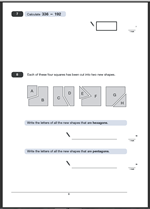Two more questions from the KS2 Maths 2010 Paper A, with answers and suggested method of answering.

The first question is a straightforward subtraction calculation. It is worth one mark and no extra marks are given for showing the working out. There are several ways of doing this: I would do it mentally by adding on from 192 to 200, which is 8.

Then going from 200 to 336 is 136.

Finally adding the 8 to the 136 making 144.

Of course, it can be done using the standard written subtraction method, or any other way that the child feels confident with.

The second question is just as straightforward.Hexagons are 6 sided shapes, but do not need to have all the sides the same length; pentagons are 5 sided shapes. Only one mark for each question and all the shapes need to be identified to gain the mark ie B and F for 8a. and C, D and G for 8b.

Questions 7 and 8 Paper A 2010

Questions 7 and 8 Paper A answers